Degree & Coefficient of a polynomial

Chapter 2 Class 9 Polynomials (Term 2)
Concept wise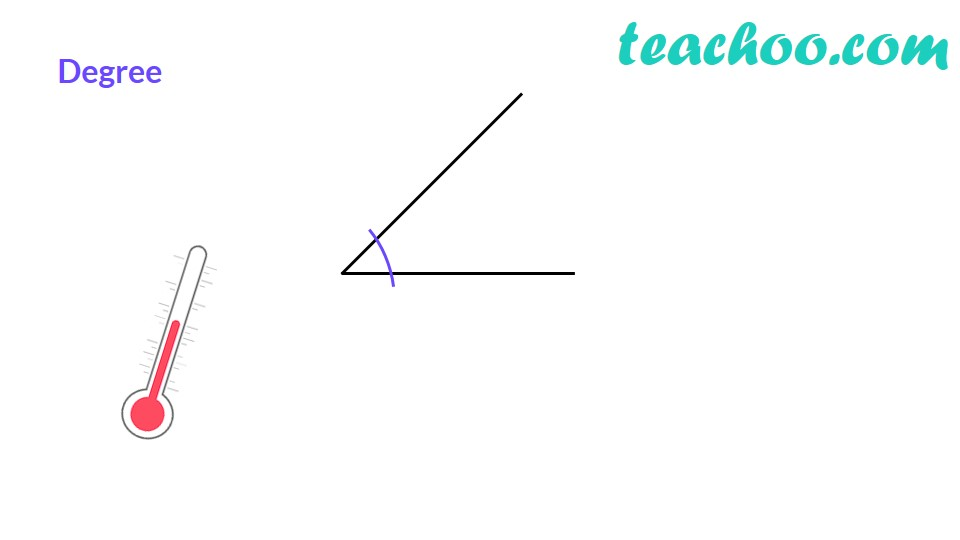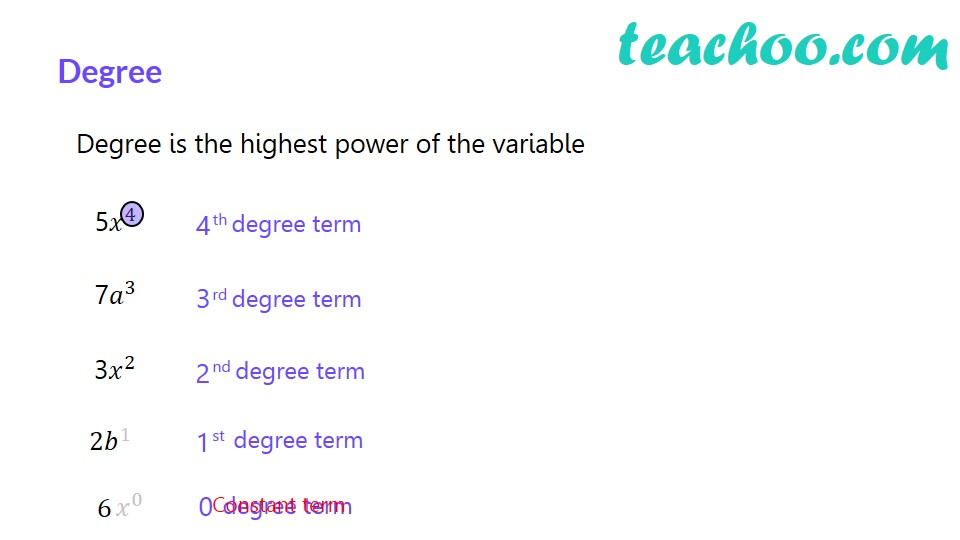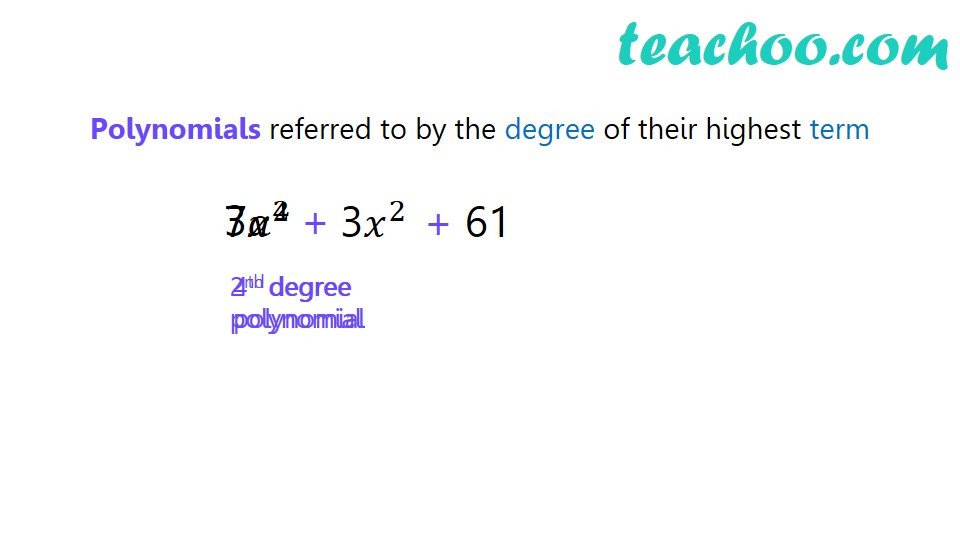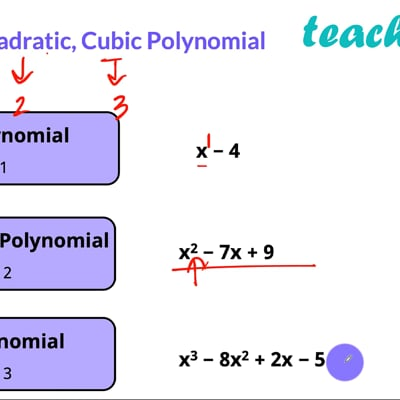This video is only available for Teachoo black users

Highest power of a variable in a polynomial is the degree of a polynomial.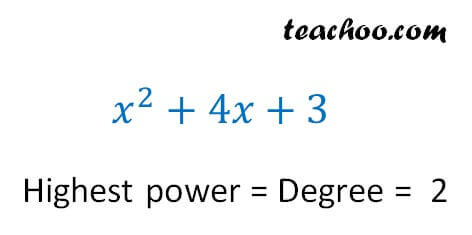Eg:- x 3 − 3x 2 + 4x + 10

Degree = Highest power = 3

Eg:-   30x 4 + 5x 3  − 10x 5 + 41

Degree = Highest power = 5

### Transcript

Degree!Degree Degree is the highest power of the variable th degree term rd degree term nd degree term st degree term Constant term Why is degree so important?Polynomials referred to by the degree of their highest term3𝑎^2 3𝑥^2 61 2nd degree polynomial